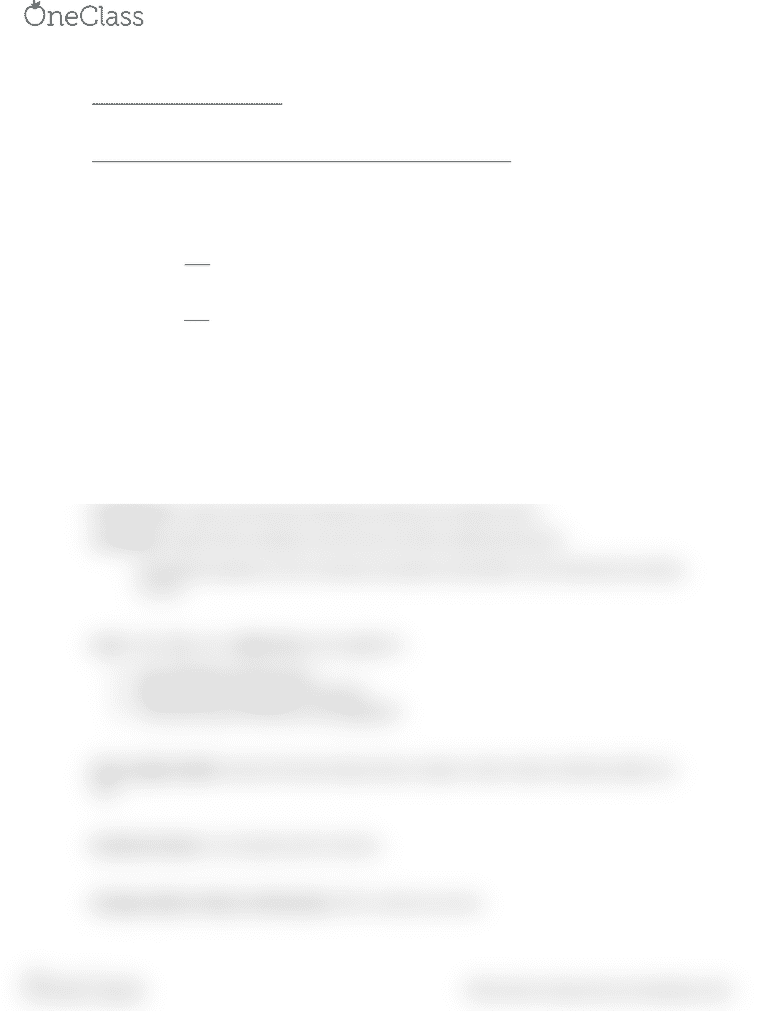Textbook Notes (270,000)
US (100,000)
JMU (200)
COB (90)
COB 241 (10)
Chapter 5

COB 241 Chapter Notes - Chapter 5: Inventory Turnover, Gross Margin, Financial Statement

Department
Course Code
COB 241
Professor
James Irving
Chapter
5

This preview shows half of the first page. to view the full 2 pages of the document.Ch. 5: Accounting for Inventories
Four acceptable methods for determining the amount of cost to transfer:
1. Special Identification
a. costs of goods sold would be \$100 if the first item purchased was sold or \$110 if the
second item purchased was sold
2. First in, First out
a. FIFO
b. Requires that the cost of the items purchased first be assigned to the cost of goods sold
3. Last in, First out
a. LIFO
b. Requires that the cost of the items purchased last be charged to cost of goods sold
4. Weighted Average
a. Calculate the average cost per unit by dividing the total cost of the inventory available
by the total number of units available.
b. Cost of goods sold is then calculated by multiplying the average cost per unit by the
number of units sold
Physical Flow: goods usually move physically on a FIFO basis
Full disclosure: requires that financial statements disclose the method chosen
Consistency: requires that companies use the same cost flow method each period
The limited exceptions to the consistency principle are described in more advanced accounting
courses
Lower of cost or market rule can be applied to:
1. Each individual inventory item
2. Major classes or categories of inventory
3. The entire stock of inventory in the aggregate
Gross margin method: assumes the percentage of gross margin to sales remains relatively stable over
time
Inventory turnover: cost of goods sold / inventory
Average number of days to sell inventory: 365 / inventory turnover
find more resources at oneclass.com
find more resources at oneclass.com